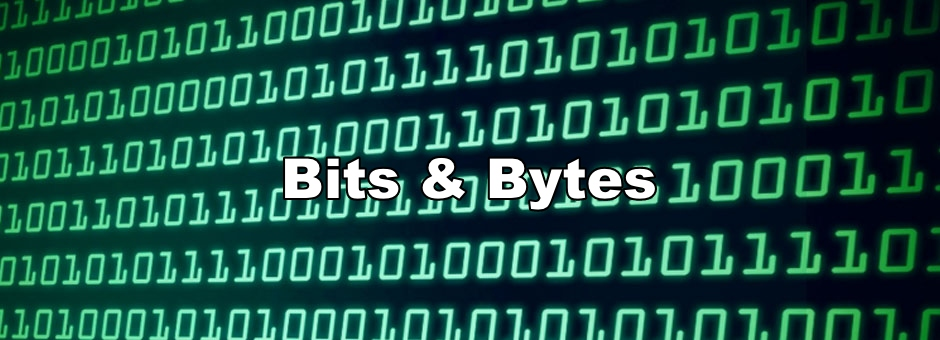Yesterday, we talked about how the Two’s Complement scheme has caused numerous issues with regards to overflow. Today, we’re going to figure out how to deal with overflow and then move onto exploring issues regarding floats and doubles. How do we avoid overflow & underflow? Unfortunately, there’s no one size fits all approach when dealing with overflow and underflow issues. Each language handles this problem differently. Some popular languages like Java have built in classes like BigInteger where clients can specify a string for the very large number that they want to represent. Here’s an example:BigInteger behind the scenes keeps a set of bits of arbitrary length so you can easily express numbers that are far beyond the limits of a long. Other languages like Ruby and Python automatically convert integers to their BigInteger counterparts behind the scenes so you as the programmer won’t have to deal with overflow. Pretty neat! While underflow and overflow are generally not desirable behaviors, it’s worth noting there are some instances where this is okay. For example, if we’re computing a hash code for an object, having overflow or underflow won’t make a difference since the integer computed is used to interact with hashing in hash tables. The bottom line is that when dealing with very small or large numbers, also be aware of what built in language features or classes are available to you as the programmer to avoid unwanted outcomes. Sounds good! So now how do we represent floats & doubles? Just like ints and longs, floats and doubles suffer from similar issues. Floats are and doubles represent fractional values and are susceptible to over and underflow. Floats are represented with 32 bits and doubles with 64 bits. The official standard explaining how floats and doubles are represented spans 58 pages long, so we won’t be diving deep into the intricacies of how floating point types are represented. Instead, we’ll take a look at the floating point number representation to help illustrate the limitations with these types. Below is the anatomy of a float represented by 32 bits: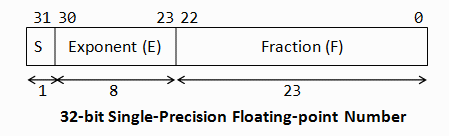In this photo: S represents the signed bit, either 0 or 1, negative or positive E represents the exponent. This represents the magnitude of the fractional component F represents the fractional component Like I mentioned, we won’t be going into too many details for floating point representation since it’s not all too important to explore the different issues. One of the first issues with this scheme is the fact that just like ints and longs, there’s a fixed number of bits to represent the fractional portion of the float. As a result, the precision of a float is limited. Notice what happens when we try to represent a large fractional number in the Java: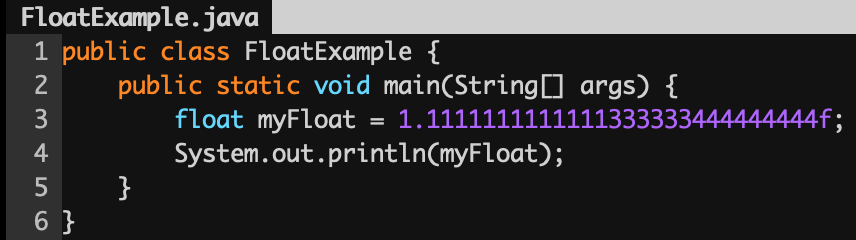The result: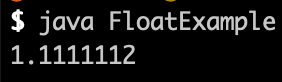Notice that the actual float got rounded up which shows that there is a limit when trying to represent precise floating point numbers. The complexities with floating point numbers start to shine when trying to do mathematical operations. It turns out that floating point addition and subtraction is not associative: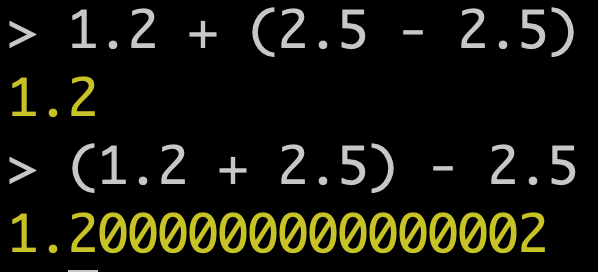Which makes floating point numbers less than ideal when trying to compute very small values. How do we deal with inaccuracies with floating point representation? A simple way to avoid being burned by floats and doubles is to not make use of the types at all. Let’s say now we want to represent products we were selling in our Amazon store in our database. We could easily represent the price with a double. However, if the customer is buying a lot of items, we might run into issues with rounding which could lead to some accounting issues down the line. Instead, we could make use of one integer to represent the number of dollars and another integer to represent the number of cents. This way, we can avoid having to deal with rounding issues all together. If we needed to use fractional numbers, some languages provide classes that will allow us to represent small numbers. In Java, the BigDecimal class (similar to BigInteger) provides us with just that: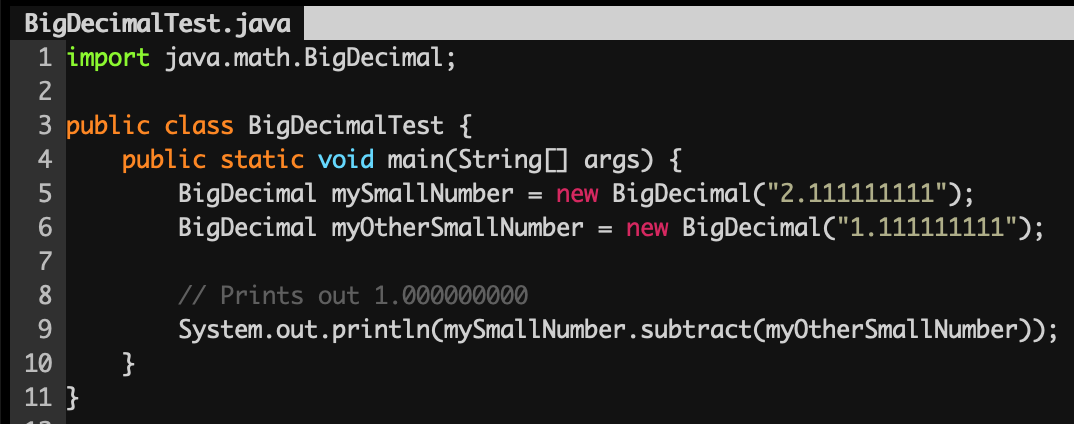Floating point equality One last thing to point out before we close it for today is that we should also be careful when trying to compare floats for equality. As a result of rounding issues, two floating point numbers might be very close, but not exactly equal. When comparing floats, it's best practice to take the absolute value of the difference between the two numbers and ensure it falls within some small delta: ```if (Math.abs(myFloatA - myFloatB) < 0.0001) {     // Then they’re “equal” }``` Final words It’s important to understand the fundamentals about how a computer represents data and by understanding how numbers are represented under the hood, we can get sense about how to avoid a certain class of mistakes. While it might seem like there are lots of limitations with representing numbers, in the coming days, we’ll see how we can leverage bits & bytes to construct one of the most space efficient data structures (that's useful for interviews too!), the BitSet. As always, below is a link to the Discord. Come join to chat!Justin
 Copyright © 2021 Gestone, All rights reserved. Want to change how you receive these emails? You can update your preferences or unsubscribe from this list.